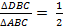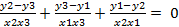Class 10 Maths Important Questions(3 Marks questions) for Coordinate Geometry

Given below are the Class 10 Maths Important Questions(5 Marks questions) for Coordinate Geometry
a) Concepts questions
b) Calculation problems
c) Multiple choice questions
d) Long answer questions
e) Fill in the blank's
1) Determine the ratio in which the point P (m, 6) divides the join of A (-4, 3) and B (2, 8). Also find the value of m.
2) The line segment joining the points (3, -4) and (1, 2) is trisected at the points P and Q. If the co-ordinates of P and Q are (p, -2) and (5/3, q) respectively. Find the values of p and q.
3) The line joining the points (2, 1) and (5, -8) is trisected at the points P and Q. If point P lies on the line 2x – y + K = 0. Find the value of K.
4) A (4, 2), B (6, 5) and C (1, 4) are the vertices of Δ ABC.
i)The median from A meets BC in D. Find the co- ordinates of the point D.
ii)Find the co- ordinates of point P on AD such that AD : PD = 2 : 1
iii)Find the co- ordinates of the points Q and R on medians BE and CF respectively such that BQ : QE = 2 : 1 and CR : RF = 2 : 1
iv) What do you observe?
5) If the points A (6, 1), B (8, 2), C (9, 4) and d (k, p) are the vertices of a parallelogram taken in order, then find the value of k and p.
6) Two vertices of a triangle are (1, 2), (3, 5) and its centroid is at the origin. Find the co-ordinates of the third vertex.
7) If C be the centroid of a triangle PQR and X be any other point in the plane, prove that
XP2+ XQ2 + XR2= CP2+CQ2+CR2+3CX2
8) If P(x, y) is any point on the line joining the points A (a, 0), B (0, b), then show that x/a + y/b = -1 9) If (-2, 3) (4, -3) and (4, 5) are the mid points of the sides of a triangle, find the co-ordinates of its centroid.
10) Fid the third vertex of a triangle, if two of its vertices are at (-3, 1) and (0, -2) and the centroid is at the origin.
11) A (3, 2) and B (-2, 1) are two vertices of a triangle ABC whose centroid G has the co- ordinates [ 5/3,-1/3 ]. Find the co- ordinates of the third vertex C of the triangle.
12) If D, E and F are the mid points of sides BC, CA and AB respectively of Δ ABC, then testing co- ordinate geometry prove that
Area of Δ DEF = (1/4)  (Area of Δ ABC)
13) If A (4, 6), B (3, -2) and C (5, 2) are the vertices of Δ ABC, then verify the fact that a median of a triangle ABC divides it into two triangles of equal areas.
14) Prove that the points (a, b + c), (b, c + a) and (c, a + b) are collinear.
15) For what value of x will the points (x, -1), (2, 1) and (4, 5) lie on a line?
16) If the points (p, q) (M, n) and (p – m, q –n) are collinear, show that pn = qm.
17) Find k so tht the point P (-4, 6) lies on the line segment joining A (k, 10) and B (3, -8). Also, find the ratio in which P divides AB.
18) Find the area of the quadrilaterals, the co- ordinates of whose vertices are
i)(-3, 2), (5, 4), (7, -6) and (-5, -4)
ii)(1, 2), (6, 2), (5, 3) and (3, 4)
iii) (-4, -2), (-3, -5), (3, -2), (2, 3)
19) Show that the following sets of points are collinear
i) (2, 5), (4, 6) and (8, 8)
ii) (1, -1), (2, 1) and (4, 5)
20) The area of a triangle is 5. Two of its vertices are (2, 1) and (3, 2). The third vertex lies on y = x + 3. Find the third vertex.
21) Four points A (6, 3), B (-3, 5), C (4, -2) and D (x, 3x) are given in such a way thatfind x.

22) If three points (x1, y1), (x2, y2), (x3, y3) lie on the same line, prove that23) If  the point P (m, 3) lies on the line segment joining the points A [-2/5, 6] and B (2, 8), find the value of m.
24) If R (x, y) is a point on the line segment joining the points P (a, b) and Q (b, a) then prove that x + y = a + b
25) Find the value of a for which the area of the triangle formed by the points A (a, 2a), B (-2, 6) and C (3, 1) is 10 square units.
26) If G be the centroid of a triangle ABC, prove that
AB2 + BC2 + CA2 = 3(GA2+ GB2+ GC2)
27.  Find the co-ordinates of circumcenter of a ? ABC where A( 1, 2) ,B ( 3, –4) and C ( 5, –6 )
28.  The coordinates of the mid-point of the line joining the points (2p + 2, 3) and (4, 2q + 1) are (2p, 2q). Find the value of p and q.
1. 3 : 2, m = (-2)/5
2. p = 7/3, q = 0
3. K = -8
4. (i)   [  7/2,9/2 ]
(ii)   [11/3,11/3  ]
(iii)  [ 11/3,11/3  ]
5. k = 7, p = 3
6. (-4, -7)
9. [ 2, 5/3  ]
10. (3, 1)
11. (4, -4)
15. x = 1

Reference Books for class 10

Given below are the links of some of the reference books for class 10 math.

You can use above books for extra knowledge and practicing different questions.

Practice Question

Question 1 What is $1 - \sqrt {3}$ ?
A) Non terminating repeating
B) Non terminating non repeating
C) Terminating
D) None of the above
Question 2 The volume of the largest right circular cone that can be cut out from a cube of edge 4.2 cm is?
A) 19.4 cm3
B) 12 cm3
C) 78.6 cm3
D) 58.2 cm3
Question 3 The sum of the first three terms of an AP is 33. If the product of the first and the third term exceeds the second term by 29, the AP is ?
A) 2 ,21,11
B) 1,10,19
C) -1 ,8,17
D) 2 ,11,20

Note to our visitors :-

Thanks for visiting our website.
DISCLOSURE: THIS PAGE MAY CONTAIN AFFILIATE LINKS, MEANING I GET A COMMISSION IF YOU DECIDE TO MAKE A PURCHASE THROUGH MY LINKS, AT NO COST TO YOU. PLEASE READ MY DISCLOSURE FOR MORE INFO.